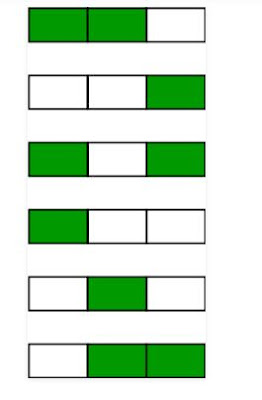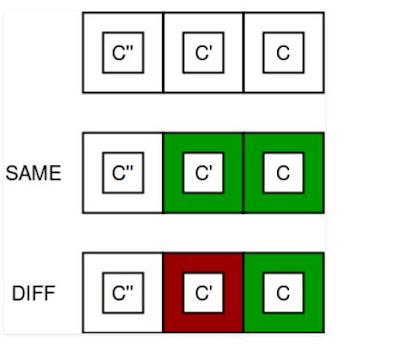## Wednesday, May 18, 2022

### Easy_Question20 : Painting Fence

There is a fence with n posts, each post can be painted with one of the k colors.

find out the number of ways of painting the fence such that at most 2 adjacent posts have the same color.

Note: n and k are non-negative integers.

Examples:

```Input : n = 2 k = 4Output : 16We have 4 colors and 2 posts.Ways when both posts have same color : 4 Ways when both posts have diff color :4(choices for 1st post) * 3(choices for 2nd post) = 12Input : n = 3 k = 2
Output : 6```
`Following image depicts the 6 possible ways of painting 3 posts with 2 colors`
```Consider the following image in which c, c’ and c” are respective colors of posts i, i-1, and i -2.the total number of ways of painting the two fences with same and different colours = k (same) + k*(k-1) (different).```public class Solution {
public static int numWays(int n, int k) {
if (n == 0)
return 0;
int same = 0, diff = k;
int res = same + diff;
for (int i = 2; i <= n; ++i) {
same = diff;
res = same +  res * (k - 1);

}
return res;
}
public static void main(String[] args)
{
int n = 3, k = 2;
System.out.println(numWays(n, k));
}
}``````

## You may also like

Kubernetes Microservices
Python AI/ML
Spring Framework Spring Boot
Core Java Java Coding Question
Maven AWS